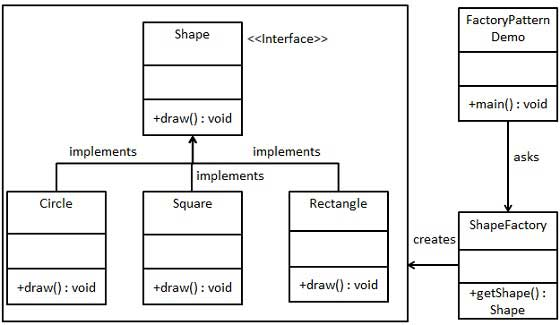# Design Pattern - Factory Pattern

#### Sequential Circuit Design

30 Lectures 3 hours

#### Professional Logo Design

81 Lectures 7.5 hours

#### PCB Design Crash Course: 3D Package & Board Design Approach

10 Lectures 3.5 hours

Factory pattern is one of the most used design patterns in Java. This type of design pattern comes under creational pattern as this pattern provides one of the best ways to create an object.

In Factory pattern, we create object without exposing the creation logic to the client and refer to newly created object using a common interface.

## Implementation

We're going to create a Shape interface and concrete classes implementing the Shape interface. A factory class ShapeFactory is defined as a next step.

FactoryPatternDemo, our demo class will use ShapeFactory to get a Shape object. It will pass information (CIRCLE / RECTANGLE / SQUARE) to ShapeFactory to get the type of object it needs.## Step 1

Create an interface.

Shape.java

```public interface Shape {
void draw();
}
```

## Step 2

Create concrete classes implementing the same interface.

Rectangle.java

```public class Rectangle implements Shape {

@Override
public void draw() {
System.out.println("Inside Rectangle::draw() method.");
}
}
```

Square.java

```public class Square implements Shape {

@Override
public void draw() {
System.out.println("Inside Square::draw() method.");
}
}
```

Circle.java

```public class Circle implements Shape {

@Override
public void draw() {
System.out.println("Inside Circle::draw() method.");
}
}
```

## Step 3

Create a Factory to generate object of concrete class based on given information.

ShapeFactory.java

```public class ShapeFactory {

//use getShape method to get object of type shape
public Shape getShape(String shapeType){
if(shapeType == null){
return null;
}
if(shapeType.equalsIgnoreCase("CIRCLE")){
return new Circle();

} else if(shapeType.equalsIgnoreCase("RECTANGLE")){
return new Rectangle();

} else if(shapeType.equalsIgnoreCase("SQUARE")){
return new Square();
}

return null;
}
}
```

## Step 4

Use the Factory to get object of concrete class by passing an information such as type.

FactoryPatternDemo.java

```public class FactoryPatternDemo {

public static void main(String[] args) {
ShapeFactory shapeFactory = new ShapeFactory();

//get an object of Circle and call its draw method.
Shape shape1 = shapeFactory.getShape("CIRCLE");

//call draw method of Circle
shape1.draw();

//get an object of Rectangle and call its draw method.
Shape shape2 = shapeFactory.getShape("RECTANGLE");

//call draw method of Rectangle
shape2.draw();

//get an object of Square and call its draw method.
Shape shape3 = shapeFactory.getShape("SQUARE");

//call draw method of square
shape3.draw();
}
}
```

## Step 5

Verify the output.

```Inside Circle::draw() method.
Inside Rectangle::draw() method.
Inside Square::draw() method.
```